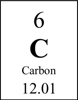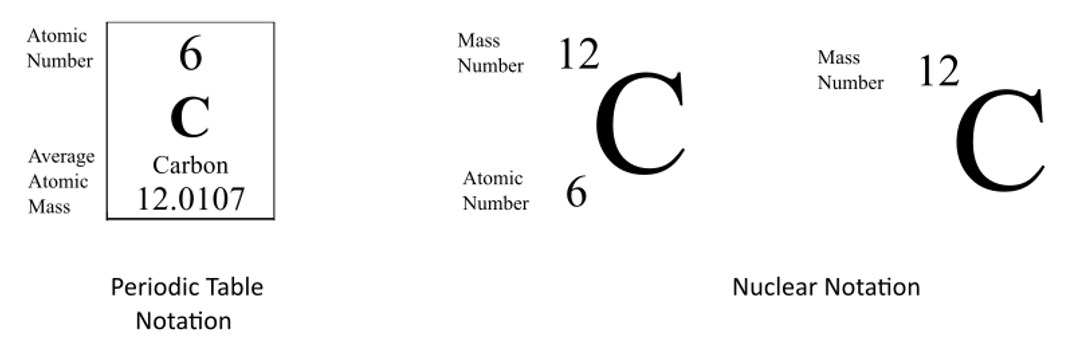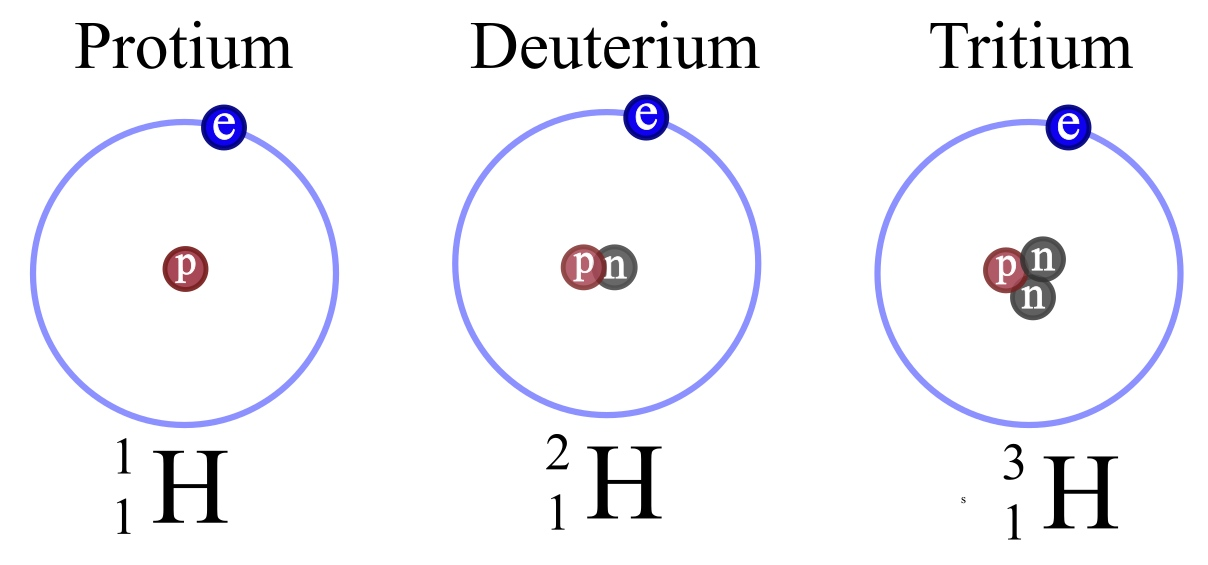# Notation and Naming (AZE notation)

In chemistry naming and notation are essential for clear communication. There are three common ways we can represent an element.

 Periodic Table Hyphen Notation Nuclear NotationCarbon-12 or C-12 (6 neutrons) $$^{12}_{6}C$$ Carbon-13 or C-13 (7 neutrons) $$^{13}_{6}C$$ Carbon-14 or C-14 (8 neutrons) $$^{14}_{6}C$$

Note: in hyphen notation, the number after the hyphen is the mass number (protons + neutrons).

For the Periodic Table, the Atomic Number is on top and the average atomic mass is on the bottom.

For nuclear notation, the mass number of the isotope goes on top and the atomic number goes on the bottom.Sometimes the Atomic Number is omitted from the nuclear notation since we already know Carbon has six protons from the atomic number on the periodic table.

Exercise: Write the Nuclear Notation for the three stable isotopes of Neon which have 10, 11, and 12 neutrons.

 imgage Ne Neon-20 Neon-21 Neon-22

 Exercise: Given the hyphen notation, write the nuclear notation. Refer to the periodic table as needed. Chlorine-34, Li-7, and H-2

Hydrogen is often given as an example of isotopes. Note that the three stable isotopes of Hydrogen are given special names. No other isotopes have special names.Protium is the only isotope that exists without any neutrons. It has one proton and one electron.

 Exercise 3: How is Hydrogen different from other elements?

Next Up: Isotopes vs Ions